Applied and Computational Mathematics
Volume 4, Issue 3, June 2015, Pages: 207-213

Some Convalescent Methods for the Solution of Systems of Linear Equations

M. Rafique, Sidra Ayub

Department of Mathematics, Faculty of Science, HITEC University, Taxila, Pakistan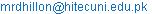(M. Rafique)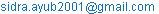(S. Ayub)

M. Rafique, Sidra Ayub. Some Convalescent Methods for the Solution of Systems of Linear Equations. Applied and Computational Mathematics. Vol. 4, No. 3, 2015, pp. 207-213. doi: 10.11648/j.acm.20150403.23

Abstract: In a variety of problems in the fields of physical sciences, engineering, economics, etc., we are led to systems of linear equations, Ax = b, comprising n linear equations in n unknowns x1, x2, …, xn, where A = [aij] is an nxn coefficient matrix, and x = [x1 x2 . . .xn]T, b= [b1 b2 . . .bn]T are the column vectors. There are many analytical as well as numerical methods1}– 11 to solve such systems of equations, including Gauss elimination method, and its modifications namely Doolittle’s method, Crout’s method and Cholesky’s method, which employ LU-decomposition method, where L = [iij] and u = [uij] are the lower and upper triangular matrices respectively. The LU-decomposition method was first introduced by the mathematician Alan M. Turing2-11 in 1948. Here, in this paper we have made an effort to modify the existing LU-decomposition methods to solve the above mentioned system Ax = b, with the least possible endeavour. It may be seen that the Gauss elimination method1, 2, 3, 4 needs about 2n3/3 operations, while Doolittle’s and Crout’s methods require n2 operations. Accordingly, in these methods we are required to evaluate n2number of unknown elements of the L and U matrices. Moreover, Cholesky’s method1 requires 2n2/3 operations. Accordingly this method requires evaluation of 2n2/3 number of unknown elements of the L and U matrices But, in contrast, the improved Doolittle’s, Crout’s and Cholesky’s methods presented in this paper require evaluation of only (n–1)2 number of unknown elements of the L and U matrices. Moreover, an innovative method is also presented in this paper which requires evaluation of even less number of unknown elements of the L and U matrices. In this method we need to evaluate only (n–2)2 number of the said unknown elements. Thus, by employing these methods, the computational time and effort required for the purpose can substantially be reduced.

Keywords: System of Equations, Matrix, Column Vector, Decomposition, Doolittle’s Method, Crout’s Method, Cholesky’s Method

1. Introduction

There are numerous analytical and numerical methods for the solution of a linear system, Ax = b, including Gauss elimination method, and its modifications namely Doolittle’s, Crout's and Cholesky’s methods. In the Doolittle’s method LU-decomposition of an nxn matrix A is of the form A = LU, which is given as under,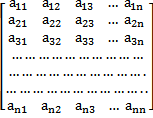=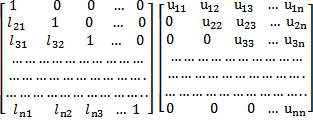.

In the case of Crout’s method this decomposition is given as,=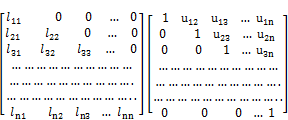It may be mentioned that both the above quoted decompositions lead to the same solution of any linear system of equations.

For a symmetric and positive definite coefficient matrix, the LU-decomposition by the Cholesky’s method is carried out as under,=The above mentioned usual LU-decomposition methods are well discussed in the books on numerical linear algebra. In these methods the coefficient matrix A is decomposed as A = LU, so that the linear system of equations Ax = b can be written as LUx = b. This equation may further be written as, Ly = b, where, Ux = y. First we solve the former equation for y by forward substitution and then solve the latter for x by backward substitution. Accordingly, we obtain the solution to the given system of linear equations.

For numerical stability, pivoting is very essential unless the coefficient matrix is diagonally dominant or symmetric and positive definite. Therefore, in all the methods discussed in this paper, pivoting has been implemented before carrying out further steps.

2. Improved Doolittle’s Method

The usual Doolittle’s method, mentioned above, was devised by the American mathematician Myrick Hascall Doolittle (1830-1913)1, 3, 7–12, which appeared in U.S. Coast and Geodetic Survey in 1878. Here, in our improved method, we consider a linear system of n equations in n unknowns, Ax = b. To start with pivoting is done to ensure numerical stability. Thereafter, the system of linear equations is transcribed in such a way that the pivot equation is made to have the pivot element as unity, i.e., a11 = 1. For this purpose, if required, we may have to select the requisite equation and divide it throughout by the coefficient of x1 for the needful, and make it the pivot equation. Following these steps, the main considerations for the decomposition of the coefficient matrix A, as A = LU, are as under:

(i) The first column of the matrix L = [lij] is kept the same as that of matrix A, i.e., l­i1 = ai1, for all i.

(ii) The first row of the matrix U = [uij] is also kept the same as that of the matrix A, i.e., u1j = a1j, for all j.

(iii) The diagonal elements of the matrix L= [lij] are kept as unity, i.e., lii = 1 for all i.

Accordingly, we decompose the coefficient matrix A = LU as,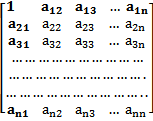=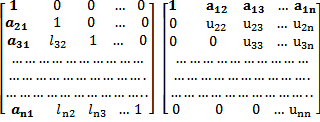Here all aij are known while lij and u­ij are unknown which are to be evaluated. For this purpose we multiply the right hand side matrices L and U, and equate the corresponding elements on both sides, i.e., set aij = (lu)ij . This correspondence enables us to evaluate all the unknown elements lij and u­ij. Here we see that for an nxn order coefficient matrix A, it is required to evaluate only (n–1)2 number of unknown elements lij, and uij of the L and U matrices respectively. It may be appreciated that by following the above mentioned scheme of LU-decomposition, the computational effort is substantially reduced. As described above, after having carried out the above mentioned decomposition, we solve Ly = b for y, followed by solution of Ux = y for x, to get the required solution to the given system of equations. Here we discuss few examples to illustrate the above mentioned method.

2.1. Solution of a System of Three Equations in Three Unknowns

We consider the following system of equations,

5x1 + x2 + 2x3 = 19

6x1 + 24x2 – 12x3 = –12

2x1 + 3x2 + 8x3 = 39

We interchange 1st and 2nd equations and then divide the pivot equation by 6 so as to have a11 = 1, as required by the method explained above. Then we decompose the coefficient matrix as A = LU, according to the scheme described above. Here the coefficient matrix A is of order 3x3, so we need to find only (3–1)2 = 4 number of unknown elements of the L and U matrices, which turn out as, l32 = 0.26, u22 = –19, u23 = 12, u33 = 8.84. Thus the coefficient matrix gets decomposed as A = LU,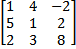=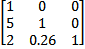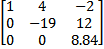Having carried out the above decomposition, we solve Ly = b and obtain y = [–2 29 35.37]T. Next solving Ux = y we get the solution to the given system of equations as,

x = [2 1 4]T

2.2. Solution of a System of Five Equations in Five Unknowns

We consider the following system of linear equations,

5x1 + 10x2 + 15x3 – 20x4 + 5x5 = – 20

2x1 + x2 + 5x3 + 3x4 + 2x5 = –2

3x1 + 4x2 + x3 + 2x4 + 4x5 = 4

4x1 + x2 + 3x3 + 5x4 + x5 = 6

2x1 + 4x2 – x3 + 2x4 + 5x5 = 3

We divide the pivot equation by 5 in order to make a11 = 1, and then decompose the coefficient matrix as described above and obtain (5–1)2 = 16 unknown elements of the L and U matrices as, l32 = 0.67, l42 = 2.33, l32 = 0, l43 = 0.91, l53 = 0.95, l54 = – 0.34, and, u22 =–3, u23 = –1, u24 = 11, u25 = 0, u33 = – 7.33, u34 = 6.67, u35 = 1, u44 = – 10.73, u45 = – 3.91, u55 = 0.72. Accordingly, we obtain,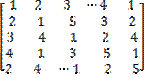=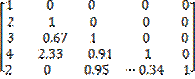x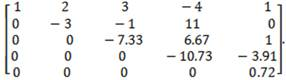After having carried out the above mentioned decomposition, we solve Ly = b for y, where, y = [y1 y2 y3 y4 y5]T, and b = [– 4 –2 4 6 3]T. Thus we obtain, y = [–4 6 12 –2.91 – 1.44]T. Thereafter, solving Ux = y for x, we get the solution to the given system of equations as, x = [1 2 –1 1 2]T.

3. Improved Crout’s Method

The standard Crout’s method was formulated by the American mathematician Prescott Durand Crout (1907 – 1984)6–12 . As in the case of improved Doolittle’s method, here also, after having carried out pivoting, the pivot equation is divided by the coefficient of x1. Thereafter, the main idea is to write out the system Ax = b in such a way that the pivot equation has its pivot element a11 = 1. Furthermore, the main considerations for the decomposition of the coefficient matrix A, as A = LU, are as under:

(i) As in the case of improved Doolittle’s method, we keep l­i1 = ai1, for all i.

(ii) Same as in the case of improved Doolittle’s method we retain u1j = a1j, for all j.

(iii) The diagonal elements of the matrix U are kept as unity, i.e., uii = 1 for all i.

Accordingly, we decompose the coefficient matrix A = LU as,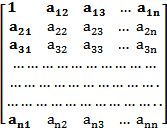=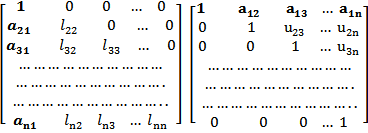Here all aij are known while lij and u­ij are unknown which are to be evaluated. For this purpose we multiply the right hand side matrices L and U, and equate the corresponding elements on both sides, i.e., set aij = (lu)ij . This correspondence enables us to evaluate all the unknown elements lij and u­ij.

We see that for an nxn order coefficient matrix A, it is required to evaluate only (n–1)2 unknown elements lij, and uij of the L and U matrices respectively. It may be appreciated that by following the above mentioned scheme of LU-decomposition, the number of operations are substantially reduced. As described above, after having carried out the above mentioned decomposition, we solve Ly = b for y, followed by Ux = y for x, to obtain the required solution to the given system of linear equations. Here we discuss few examples to illustrate the above mentioned method.

3.1. Solution of a System of Four Equations in Four Unknowns

We consider the following system of equations,

5x1 + 10x2 + 15x3 – 20x4 = –10

2x1 + x2 + 5x3 + 3x4 = 2

3x1 + 4x2 + x3 + 2x4 = 12

4x1 + x2 + 3x3 + 5x4 = 8

We divide the pivot equation by 5 to make a11 = 1, and then decompose the coefficient matrix, as illustrated above. Thus we obtain a total of (n–1)2 =(4–1)2 = 9 unknown elements of the L and U matrices as, l22 = –3, l32 = –2, l42 = –7, l33 = –7.33, l43 = –0.33, l44 = –10.73, u23 = 0.33, u24 = – 3.67, u33 = 9, u34 = –0.91. Thus the decomposition A = LU, is obtained as,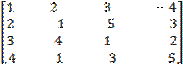=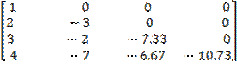x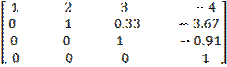Next, solving Ly = b, where b is the column vector, [–2 2 12 8]T we obtain, y = [–2 –2 –1.91 1]T, and then solving Ux = y, we get the solution to above system as x = [1 2 –1 1]T.

3.2. Solution of a System of Five Equations in Five Unknowns

We consider the following system of equations,

16x1 – 8x2 + 8x3 + 16x4 + 24x5 = 40

3x1 + 2x2 + 2x3 + 2x4 + 4x5 = 9

3x1 + 3x2 – 3x3 + 2x4 – 4x5 = 7

5x1 – x2 – 1x3 + 3x4 – 7x5 = 1

7x1 + x2 + 3x3 + 5x4 – 5x5 = 5

We divide the pivot equation throughout by 16, to make a11 = 1, and then decompose the coefficient matrix as enunciated above. Thus we obtain (n – 1)2= (5 – 1)2 = 16 unknown terms of the L and U matrices as: l22 = 3.5, l32 = 4.5, l42 = 1.5, l52 = 4.5, l33 = – 5.14, l43 = 3.71, l53 = 1.14, l44 = –1.78, l54 = – 0.78 l55 = – 9.34, u23 = 0.14, u24 = – 0.29, u34 = 0.056, u25 = – 0.14, u35 = 1.53, and, u45 = 4.84. Accordingly, we get the desired LU decomposition of the coefficient matrix as,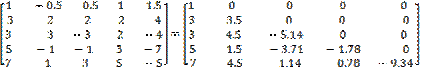x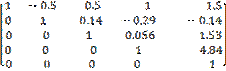Having carried out the above mentioned decomposition, we first solve Ly = b, where b = [2.5 9 7 1 5]T. It gives y = [2.5 0.43 0.47 5.84 1]T. Next, we solve Ux = y, and obtain the solution to the given system of equations as, x = [1 1 – 1 1 1]T.

4. Improved Cholesky’s Method

The usual Cholesky’s method for symmetric and positive definite coefficient matrix was developed by French military officer, geodesist and mathematician Andre-Louis Cholesky (1876–1918)1, 12 . As discussed above, for all practical algorithms pivoting is necessary unless the matrix is diagonally dominant, or symmetric and positive definite. Here, in our improved method we follow the same scheme as in the case of improved Doolittle’s method. Accordingly, we decompose the coefficient matrix A = LU as,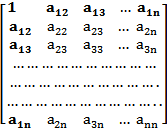=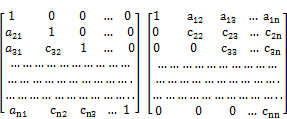Here all aij are known while cij are unknown, which are to be evaluated. For this purpose we multiply the right hand side matrices L and U, and equate the corresponding elements on both sides. This correspondence enables us to evaluate all the unknown elements cij.

We see that for an nxn order coefficient matrix A, we have to evaluate only (n–1)2 unknown elements cij of the L and U matrices respectively. It may be appreciated that by following the above mentioned scheme of LU-decomposition, the number of operations are substantially reduced. As described above, after having carried out the above quoted decomposition, we solve Ly = b for y, followed by Ux = y for x, to obtain the required solution to the given system of linear equations. Here we discuss few examples to illustrate the above mentioned method.

4.1. Solution of a System of Four Equations in Four Unknowns

We consider the following system of equations,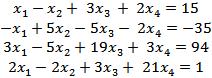Decomposing the coefficient matrix we obtain (n–1)2 =(4–1)2 = 9 unknown elements of the L and U matrices as, l32 = – 0.5, l42 = 0, l43 = – 0.33, u22 = 4, u23 = – 2, u24 = 0, , u33 = 9, u34 = – 3, u44 = 16. Thus the decomposition A = LU, is given as,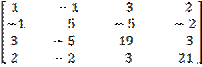=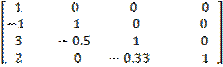x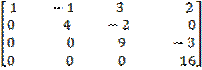Next, solving Ly = b, where b is the column vector, [15 –35 94 1]T we obtain, y = [15 –20 39 –16]T, and then solving Ux = y, we get the solution to the given system of equations as x = [2 –3 4 –1]T.

4.2. Solution of a System of Five Equations in Five Unknowns

We consider the following system of equations,

12x1 + 18x2 + 24x3 + 30x4 + 12x5 = 24

3x1 – x2 + x3 – 2x4 + x5 = 9

4x1 + x2 + 2x3 + x4 – x5 = 5

5x1 – 2x2 + x3 + 2x4 + x5 = 8

2x1 + x2 – x3 + x4 + x5 = – 1

We divide the pivot equation by 12 to set a11 = 1, as required by the method discussed in this paper. Thereafter, we decompose the coefficient matrix as, A = LU, as described above. In this case we need to evaluate (5–1)2 = 16 unknown terms of the L and U matrices as, l32 = 0.91, l42 = 1.73, l52 = 0.36, l43 = 0.25, l53 = 2.19, l54 = 0.042, and u22 = – 5.5, u23 = –5, u24 = – 9.5, u25 = –2, u33 = – 1.45, u34 = –0.36, u35 = – 3.18, u44 = 6, u45 = 0.25, u55 = 6.68. This decomposition is given as,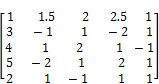=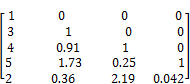x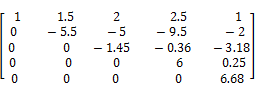After carrying out the above mentioned decomposition, first we solve Ly = b, where b = [2 9 5 8 –1]T, to get y = [2 3 –5.73 –5.75 6.68]T. Thereafter, we solve Ux = y, and obtain the solution to the given system of equations as,

x = [1 –1 2 –1 1]T.

5. An Innovative LU-Decomposition Method

We consider a linear system of n equations in n unknowns, Ax = b, where A is the coefficient matrix of order nxn, while x and b are column vectors of order nx1, each. First of all we carry out pivoting unless the coefficient matrix is diagonally dominant or symmetric and positive definite. This method necessitates to have the coefficients of x1 in all the equations as unity, i.e., ai1 = 1 for all i. Any equation not fulfilling this condition may be divided throughout by the coefficient of x1 for the needful. Thereafter, we can decompose the coefficient matrix as, A = LU, with the following provisions,

(i)         The first column of the lower triangular matrix L = [lij] is kept the same as that of matrix A, i.e., li1= ai1 = 1, for all i.

(ii)       The first row of the upper triangular matrix U = [uij] is also kept the same as that of matrix A, i.e., u1j = a1j, for j ≥ 2. The elements of the second column of matrix L are transformed as, li2 = ai2 a12,

(iii)      for i ≥ 2.

(iv)     The diagonal elements of the matrix U are kept as unity, i.e., uii =1, for all i.

(v)       The remaining elements in the second row of matrix U are transcribed as: u2j =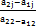for j ≥ 3.

Thus, schematically, LU-decomposition of the coefficient matrix A = LU, is given as under,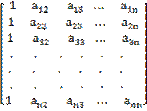=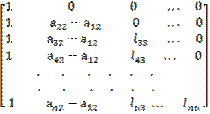x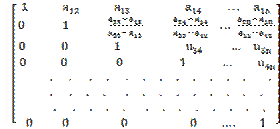After having accomplished the LU-decomposition as described above, we solve the equation Ly = b for y and next solve the equation Ux = b for x, to obtain the solution to the given system of linear equations. Here we consider few examples to elaborate the method discussed above.

5.1. Solution of a System of Three Equations in Three Unknowns

We consider the system of equations,

5x1 + x2 + 2x3 = 19

x1 + 4x2 – 2x3 = – 2

2x1 + 3x2 + 8x3 = 39.

We divide the first equation by 5 and the third equation by 2, throughout as required by the method elaborated above to get,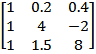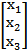=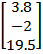Next, the coefficient matrix is decomposed as described above. In this decomposition only (n – 2)2 =

(3–2)2 = 1 number of unknown, namely l33 is required to be evaluated which comes to 4. Accordingly, we obtain the required decomposition A = LU, as,=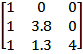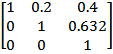.

Further, solving Ly = b, we obtain, y = [3.8 1.3 4]T. Thereafter, solving Ux = y, we get, x = ]T, which is the required solution to the given systems of equations.

5.2. Solution of a System of Four Equations in Four Unknowns

We consider the system of equations,

4x1 – 4x2 + 12x3 + 8x4 = 60

–x1 + 5x2 – 5x3 – 2x4 = – 35

3x1 – 5x2 + 19x3 + 3x4 = 94

2x1 – 2x2 + 3x3 + 21x4 = 1

We divide the pivot equation by 4, multiply the second equation by –1, divide the third equation by 3, and also divide the fourth equation by 2, as discussed above, and transform the given system of equations as Ax = b, where b = [ 15 35 31.33 0.5]. Here we need to evaluate only (n –2)2 = (4–2)2 = 4 unknown number of elements of the L and U matrices, which are obtained as: l33 = 3, l43 = – 1.50, l44 = 8, and u34 = – 0.33. Thus we get A = LU as under,=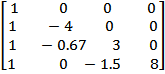x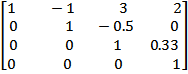After having carried out this decomposition, we solve the system Ly = b for y to get y = [15 –5 4.33 1]T. Thereafter, solving the system Ux = y, for x we obtain the solution to the given system of equations as, x = [2 –3 4 –1]T.

By following the method discussed above, we can solve a linear system having any number of equations with the same number of unknowns.

6. Conclusion

The solution of a system of linear equations by means of LU-decomposition of the coefficient matrix is a plausible method that can be employed analytically as well as numerically. It may be seen that for an nxn coefficient matrix, the usual Doolittle's, and Crout's methods require evaluation of a total of n2 number of unknown elements of the L and U matrices, and in the case of usual Cholesky’s method we are required to evaluate 2n2/3 number of unknown elements of these matrices. However, the improved Doolittle’s, Crout’s and Cholesky’s methods need evaluation of only (n –1)2 number of elements of these matrices, while the Innovative LU-Decomposition method requires evaluation of only (n –2)2 number of unknown elements of the L and U matrices. This difference becomes significant for systems of large number of linear equations. As such a considerable amount of computational time and energy can be saved by employing the methods presented in this paper.

References

1. E. Kreyszig: Advanced Engineering Mathematics, John Wiley, (2011)
2. A. M. Turing: Rounding-Off Errors in Matrix Processes", The Quarterly Journal of Mechanics and Applied Mathematics 1: 287-308.doi:10.1093/qjmam/1.1.287 (1948)
3. David Poole: Linear algebra: A Modern Introduction (2nd Edition), Thomson Brooks/Cole ISSN 0-534-99845-3 (2006)
4. James R. Bunch, and John Hopkins: Triangular factorization and inversion by fast matrix multiplication, Mathematics of Computation, 28, 231-236, doi:10.2307/2005828, ISSN 0025-5718
5. J H Wilkinson: The Algebraic Eigenvalue Problem, Oxford University Press (1988)
6. B. Faires, Numerical Analysis, PWS Pub., Boston, (1993).
7. G.H.Golub and C.F.Van Loan, Matrix Computations, John Hopkins, Baltimore, (1989).
8. J.H. Mathews and K. D. Fink: Numerical Methods Using MATLAB (4th Edition)
9. L. V. Fausett: Applied Numerical Analysis Using MATLAB, Pearson, (2009)
10. S.C.Chapra and R.P.Canale, Numerical Methods for Engineers, Mc-Graw-Hill, New York,(1990).
11. Petre Teodorescu, Nicolae-Doru Stanescu, Nicolae Pandrea: Numerical Analysis with Applications in Mechanics and Engineering, John Wiley (2013).

 Contents 1. 2. 2.1. 2.2. 3. 3.1. 3.2. 4. 4.1. 4.2. 5. 5.1. 5.2. 6.
Article ToolsAbstractPDF(1089K)